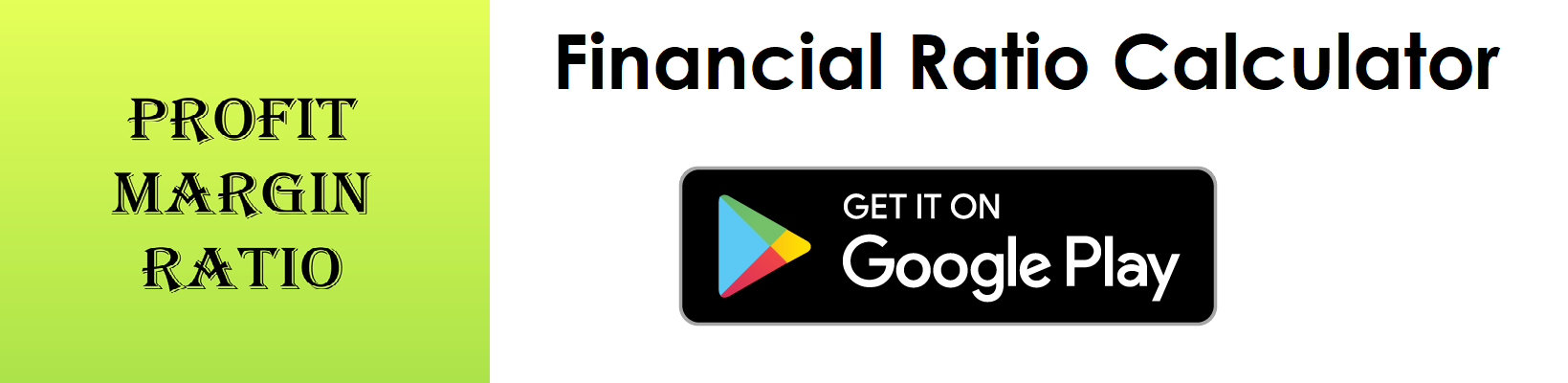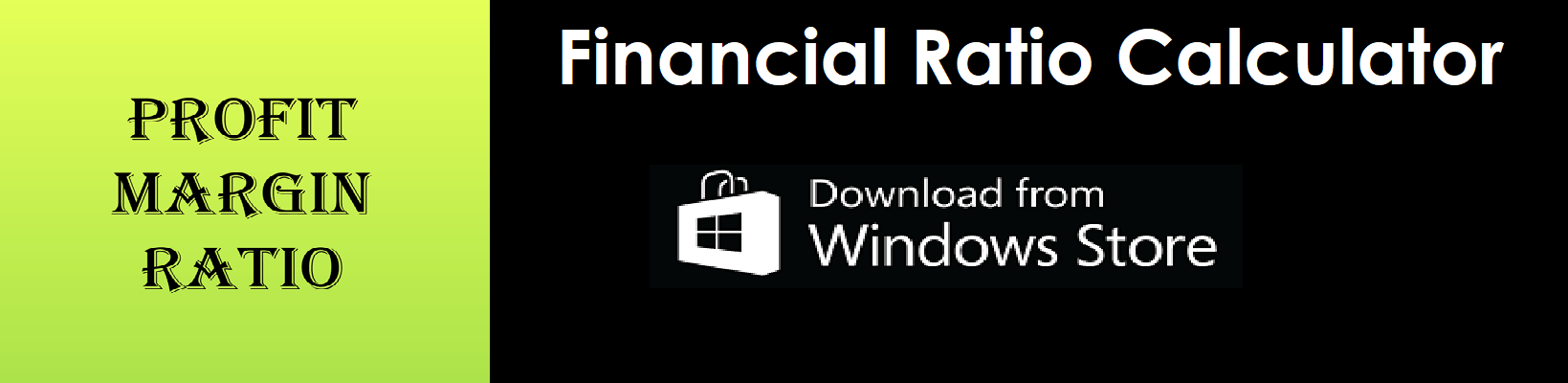# Interest Coverage Ratio Calculator

Key in the EBIT and Interest Expense Values from your Income Statement to the respective fields given below and then click Calculate to get the desired result.

##### Interest Coverage Ratio Calculator - Glossary:
Using our interest coverage ratio calculator, helps to compare, measure, understand the overall health of the business.

##### Interest Coverage Ratio:
Shows the proportion of Earnings Before Interest & Tax (EBIT) and Interest Expense. In simple words, measures a company's ability to make interest payments on its debt in a timely manner.

##### How to calculate?
Formula: Interest Coverage Ratio = Earnings Before Interest and Tax (EBIT)/Interest Expense
This is an income statement component; the values are commonly stated against EBIT and Interest Expense.

##### Earnings Before Interest & Tax (EBIT):
Profit before deducting all its interest & taxes.

##### Interest Expense:
Interest expense is the cost of debt - the amount paid for servicing the amount borrowed by the company.

##### Example:
Interest coverage ratio for a company with an earnings before interest and tax (EBIT) of \$100,000 and interest expense of \$25,000 is 4 times. It can meet its interest payments 4 times of its earnings. Interest coverage ratio lower than 1.5 times means a company is vulnerable and may face difficulties to meet its interest payments.

# Download our Mobile Apps - today.